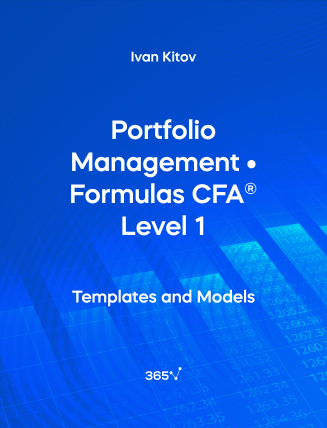## Portfolio Management • Formulas CFA® Level 1###### Ivan Kitov

Need an all-in-one list with the Portfolio Management formulas included in the CFA® Level 1 Exam? We have compiled them for you here. The relevant formulas have been organized and presented by chapter. In this section, we will consider Portfolio Risk and Return calculations.

## 1. Portfolio Management: an Overview

Diversification ratio

Diversification~ratio = \frac {σ~of~equally~weighted~portfolio~of~n~securities}{σ~of~single~security~selected~at~random}

σ = Volatility (Standard deviation)

Net asset value per share

Net~asset~value~per~share = \frac {Fund~Assets - Fund~Liabilities}{Number~of~Shares~Outstanding}

## 2. Portfolio Risk and Return: Part I

Holding Period Return (HPR) – No cash flows

HPR = \frac {Ending~value - Beginning~value}{Beginning~value}

Holding Period Return (HPR) – Cash flows occur at the end of the period

HPR = \frac {Ending~value-Beginning~value+Cash~flows~received}{Beginning~value}=\frac {P{_1} - P{_0} + D{_1}}{Beginning~value}

Holding Period Return (HPR) – Multiple years

HPR = [(1 + R{_1}) \times (1 + R{_2})] - 1

R{_1} = Holding period return in year 1
R{_2} = Holding period return in year 2

Arithmetic mean return

\overline {R{_i}}=\frac {R{_{i1}}+R{_{i2}}+…+R{_{iT-1}}+R{_{iT}}}{t}=\frac {1}{T}\sum_{t=1}^TR{_{it}}

overline {R{_i}} = Arithmetic mean return
R{_{it}} – Return in period t
T = Total number of periods

Geometric mean return

\overline {R{_{Gi}}}=\sqrt {(1+{R{_{i1}})\times (1+{R{_{i2}}) \times…\times  (1+R{_{i,T-1}}) \times (1+R{_{i,T})}}}}-1=\sqrt [T]{ \prod_{t=1}^T(1+R{_{it})}}-1

Internal Rate of Return (IRR)

\sum_{t=0}^N \frac {CF_t}{(1+IRR)^t}=0

t = Number of periods
CF_t = Cash flow at time t

Time-weighted rate of return

r{_{TW}} = \big[(1 + r_1) \times (1 + r_2) \times ... \times (1 + r_N)\big]^{\frac {1}{N}}-1

r_{N} = Holding period return in year n

Annualized return

r_{annual} = (1 + r_{period})^c - 1

r = Periodic return
c = Number of periods in a year

Nominal rate of return

(1 + r) = (1 + r_{rF}) \times (1 + π) \times (1 + RP)

r_{rF} = Real risk-free rate of return
π = Inflation

Real rate of return

(1 + r_{real}) = (1 + r_{rF}) \times (1 + RP) = \frac {(1+r)}{(1+π)}

r_{rF} = Real risk-free rate of return
π = Inflation

Population variance

σ^2=\frac {\sum_{i=1…n}^N (x_i-μ)^2}{N}

x_{i} = Return for period i
N = Total number of periods
μ = Mean

Population standard deviation

\sqrt {σ=\frac {\sum_{i=1…n}^N (x_i-μ)^2}{N}}

x_{i} = Return for period i
N = Total number of periods
μ = Mean

Sample variance

S^2=\frac {\sum_{i=1…n}^n(x_i-\overline x)^2}{n-1}

X_i = Return for period i
N = Total number of periods
\overline x = Mean of n returns

Sample standard deviation

\sqrt {s=\frac {\sum_{i=1…n}^n(x_i-\overline x)^2}{n-1}}

X_i = Return for period i
N = Total number of periods
\overline x = Mean of n returns

Covariance

COV_{1,2} =\frac {\sum_{t=1}^n {[R_{t,1}-\overline {R_1}][R_{t,2}-\overline {R_2}]}}{n-1}=ρ_{1,2}σ_{1}σ_{2}

R_{T1} = Return on Asset 1 in period t
R_{T2} = Return on Asset 2 in period t
ρ = Correlation
\overline R = Mean of respective assets

Correlation

ρ_{1,2}=\frac {COV_{1,2}}{σ_{1}σ_{2}}

σ = Standard deviation

Utility function

U = E(r) - \frac {1}{2}Aσ^2


U = Utility of an investment
E(r) = Expected return
σ^2 = Variance of the investment
A = Risk aversion level

Portfolio return (Many risky assets)

R_{P} =\sum_{i=1}^Nw_iR_i~,~\sum_{i=1}^Nw_i=1

R_i = Return of asset i
w_i = Weight within the portfolio

Portfolio variance

σ^2_P = \sum_{i, j = 1}^N w_iw_jCOV (R_i, R_j)

w = Weights
R = Returns

Portfolio variance (Two-asset portfolio)

σ^2_P = w^2_1σ^2_1+w^2_2σ^2_2+2w_1w_2 COV(R_1, R_2)

Portfolio standard deviation (Two-asset portfolio)

σ_P = \sqrt {w^2_1σ^2_1+w^2_2σ^2_2+2w_1w_2 COV(R_1, R_2)}

Portfolio return of two assets (when one asset is the risk-free asset)

E(R_p) = w_1R_f + (1 - w_1)E(R_i)

R_f = Returns of respective asset
R_i = Returns of respective asset
w_1 = Weight in asset 1
1 - w_1 = w_2

Portfolio standard deviation of two assets (when one asset is the risk-free asset)

σ_P = \sqrt {w^2_1σ^2_f+(1-w_1)^2σ^2_i+2w_1(1-w_1)ρ_{1,2}σ_fσ_i}=(1 - w_1)σ_i

f = Risk-free asset
i = Asset
σ = Standard deviation
w = Weight

## Portfolio Risk And Return: Part II

Capital Asset Pricing Model (CAPM)

E(Ri) = R_F + β_i \big[E (R_M) - R_F\big]

β_i = Return sensitivity of stock i to changes in the market return
E(R_M) = Expected return on the market
E(R_M)-R_F = Expected market risk premium
R_F = Risk-free rate of interest

Capital allocation line

E(R_p) = R_f+ \Big (\frac {E (R_M) - R_f}{σ_m}\Big) \times σ_p

E(R_m) = Expected return of the market portfolio
R_f = Risk-free rate of return
σ_m = Standard deviation of the market portfolio
σ_p = Standard deviation of the portfolio P

Expected return (multifactor model)

E(Ri) - R_f = β_{i1} \times E(Factor~1) + β_{i2} \times E(Factor~2) + … + β_{ik} \times E(Factor~k)

β_{ik} = Stock i’s sensitivity to changes in the kth factor
(Factor k) = Expected risk premium for the kth factor

Beta of an asset

β_i = \frac {Cov(R_i , R_m)}{σ_m^2}=\frac {ρ_{i,m}~σ_iσ_m}{σ_m^2}=\frac {ρ_{i,m}~σ_i}{σ_m}

σ = Standard deviation
m = Market portfolio
i = Asset portfolio

Portfolio beta

β_P =\sum_{i=1}^n w_iβ_i  \sum_{i=1}^n w_i=1

Sharpe ratio

Sharpe~ratio = \frac {R_p - R_f}{σ_p}

R_p = Portfolio return
R_f = Risk-free rate of return
σ_p = Standard deviation (volatility) of portfolio return

M2 ratio

M^2~ratio = (R_p - R_f)\frac {σ_m}{σ_p} - (R_m - R_f)

m = Market portfolio

Treynor ratio

Treynor~ratio = \frac {E(R_p) - R_f}{β_p}

β_p = Portfolio beta
R_p = Portfolio return
R_f = Risk-free rate of return

Jensen’s alpha

α_P = R_p - \big[R_f + β_p (R_m - R_f)\big]

Follow the links to find more formulas on Quantitative Methods, Economics, Corporate Finance, Alternative Investments, Financial Reporting and Analysis, Equity Investments, Fixed-Income Investments, and Derivatives, included in the CFA® Level 1 Exam.Top